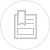# characteristic constants of gases

## gas density (table 35)

If M is the mass of a litre at 0°C, the mass of the litre at t °C for the same pressure is :

M’ is the mass of a litre at 101.3 kPa, the mass of the litre at the real pressure is :

## solubility of the main gases in water (table 36, figures 30, 31, and 32)

The following formula is used to find the amount of gas dissolved in a liquid from the Henry constant (figure 30) :

P = total gas pressure.

H = Henry constant expressed in the same unit as P.

xi = molar fraction for the gas in the liquidmolar fraction for the gas in the liquid.

yi = molar fraction for the gas in the gaseous mixture.

## kinematic viscosity of common gases

The kinematic viscosity n based on temperature, at the normal pressure of 101.3 kPa is given in m2·s–1 in table 37.

This viscosity needs to be corrected based on pressure according to the following relation (not applicable for water vapour) :

where:

' = corrected kinematic viscosity, in m2·s–1,

P' = true absolute pressure,

P = normal absolute pressure, expressed in the same unit as P'.

The density ρ' of the fluid, in kg · m–3 under temperature conditions t’ (°C) and absolute flow pressure P’ can be calculated from density r under normal conditions using the following equation :

## chlorine

### general characteristics

In its normal state, chlorine is a greenish-yellow gas; its physical constants are described in section the oxidants and disinfectants.

At 15°C and under a 101.3 kPa pressure, 1 kg of chlorine generates 314 litres of chlorine gas and 1 litre of liquid chlorine which is equal to 456 litres of gas. The gas is liquefied by cooling and by compression at a pressure that varies with temperature: 1 000 kPa at 40 °C; 500 kPa at 18 °C.

### the influence of temperature and pressure

Chlorine is a gas that irritates and suffocates; it is not corrosive in its pure and dry form. Nevertheless, it is extremely corrosive in the presence of humidity, however little. It has the following characteristics: high reactivity with most simple compounds and likely to create explosive reactions with ammonia, hydrogen…

Density (figure 35)

## ammonia

Legislation demands the use of tanks that have a 2 000 kPa safe working pressure and that have been tested at 3 000 kPa.

## ozone

### ozone solubility in water (figure 36)